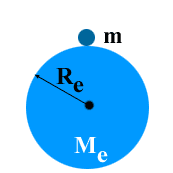# Mass Of Earth

Consider a body of mass ‘m’ placed on the surface of the earth. Let the mass of the earth is ‘Me’ and radius of the earth is ‘Re’. Neglecting the radius of body if compared with that of the earth.Gravitational force of attraction between earth and body is
F = G m Me/ Re2 ……..(1)
We know that the force of attraction of the earth on a body is equal to weight the weight of body.
i.e
F = W ……………………(2)
therefore
Comparing (1) and (2), we get,
W = G m Me/ Re2
But W = mg
mg = G m Me/ Re2
or
g = G Me/Re2
or
Me = g x Re2/G
From astronomical data:
g= 9.8 m/s2
Re = 6.38 x 106 m
G = 6.67 x 10-11 N-m2/kg2
Putting these values in the above equation.
Me = 9.8 (6.38 x 106)2/6.67 x 10-11
or